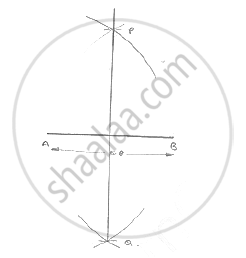# Draw a Line Segment Ab of Length 5.8 Cm. Draw the Perpendicular Bisector of this Line Segment. - Mathematics

Draw a line segment AB of length 5.8 cm. Draw the perpendicular bisector of this line
segment.

#### SolutionSteps of construction:

1. Draw a line segment AB of 5.8cm

2. With center A and radius more than 1/2 AB draw arcs with one on each side of AB

3. With center B and same radius draw arcs cutting the previous arcs at P and Q
respectively.

4. Join PQ
Hence, PQ is the perpendicular bisector of AB.

Concept: Basic Constructions
Is there an error in this question or solution?
Chapter 16: Constructions - Exercise 16.1 [Page 3]

#### APPEARS IN

RD Sharma Mathematics for Class 9
Chapter 16 Constructions
Exercise 16.1 | Q 2 | Page 3

Share